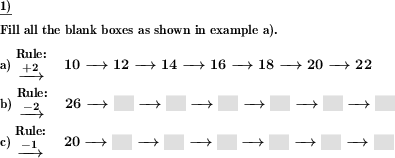Custom math worksheets at your fingertips# Details for problem "Frames & Arrows w/ elementary arithmetic - add & sub"

Quickname: 8518

Elementary School, Primary School.

## Summary

Simple frames and arrows chain task with one elementary arithmetic operation, results are to be filled in blank spaces.

## Example## Description

The task here is to enter the results in a frames and arrows style chain calculation with the operations addition and subtraction.

The calculation chain is given with a rule, a starting value, and a series of arrows with empty boxes behind them. The rule tells which operation to apply to the start value and then to each of the results. This means that the same operation must be applied in each step. For example, if the rule is "+3", then three must be added to the starting value and the result must be entered in the box following the arrow. Again add the number three to this number, and so on. Proceed in the same way when the rule specifies a subtraction task.

The number range from which all numbers originate can be chosen. The length of the calculation chain and thus the number of calculations to be performed per task can be set.

Download free printable worksheets for this math problem here. The worksheet contains the problems only, the solution sheet includes the answers. Just click on the respective link.

•Worksheet 1Solution sheet with answers
•Worksheet 2Solution sheet with answers
•Worksheet 3Solution sheet with answers

If you can not see the solution sheets for download, they may be filtered out by an ad blocker that you may have installed. If this is the case, please allow ads for this page and reload the page. The solution sheets will then reappear.

• Do these sample worksheets do not really fit?
• Do you need more math worksheets, with a different level of difficulty?
• Would you like to combine different problems on a worksheet and adjust them to your needs?
• As a teacher, you can put together your own worksheets using the automatically generated math problems provided.
With a free initial credit, you can start creating your own math worksheets in a few minutes.

You can try it for free! Register here, to create custom worksheets now!

## Customization options for this problem

Parameter
Possible values
Number of problems
1, 2, 3, 4, 5, 6, 7, 8, 9, 10
Length of chain
3, 4, 5, 6, 7
Operations
Number range
20, 30, 40, 50, 60, 70, 80, 90, 100
Sample problem
Yes, No

## Similar problems

Remark
Description
Numbers with or without decimal places have to be added.
Plus problems up to ten are to be described using marbles.
More complex form, including multiplication and division
In a frames and arrows calculation chain task, the results are to be filled in the blank spaces.
Single subtraction problem
Multiple numbers with or without decimal places have to be subtracted.
A series of marbles represents a subtraction term - empty spaces to be filled in term.Deutsche Version dieser Aufgabe
These informational pages with samples describe math problems that can be combined on custom math worksheets with solutions for home and K-12 school use.
Deutsche Seiten
×